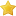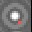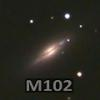## CNers have asked about a donation box for Cloudy Nights over the years, so here you go. Donation is not required by any means, so please enjoy your stay.# On calculating Signal to Noise Ratio from an image

4 replies to this topic

### #1ceteris_paribus

ceteris_paribus

Explorer 1

••• topic starter
• Posts: 89
• Joined: 21 Sep 2014
• Loc: California

Posted 13 February 2018 - 10:40 PM

So, while clouds floated by last night, I decided that I should see whether the tried and true calculations for SNR actually rang true for my camera under more real world conditions.  I found an interesting discrepancy that I hope that someone can help me with.

I took an old 30s exposure and brought it up in Pixinsight.  It was 30s, and of an open cluster, so there was really no signal to speak of that would have a low SNR, so I figured I would just calculate the noise term of the background itself based solely upon skyglow.  The skyglow would be the 'signal' and that would allow me to fill in the denominator part of the SNR formula. From what I can determine from translating the formulas to common sense, the noise term in an image should be the Standard Deviation of the image (or a sample of it).  I took a sample of the image that was devoid of stars and had about 3000 pixels in it.  I ran that through Pixinsight's statistics, and it gave me a std. dev. of about 80.  However, when calculating the expected noise in the image via: sqrt(skyglow+RN^2+darkcurrent), it came out to only 50.

I converted everything to ADU, so I don't think that it's an electron to ADU conversion problem.  I figure that either I have missed a noise term or that std. dev. of the image is not the correct way to determine the noise of an image.  I know that I number of people on this forum like to delve into this kind of stuff, so I figure that I would throw out what I've got an see if anyone has any ideas.  Also, if you calculate the SNR of an image differently, I would love to hear how you do it.

Clear skies to all,

-John

### #2freestar8n

freestar8n

Vendor - MetaGuide

•••• Posts: 8364
• Joined: 12 Oct 2007

Posted 14 February 2018 - 12:56 AM

If you have a sqrt of adu anywhere, it is probably a problem.

Best to convert everything to electrons.

Your sqrt equation implicitly assumes poisson statistics. And poisson statistics requires counts of discrete objects - like electrons. Adu’s are not counts of arriving objects. So poisson and the sqrt don’t work.

Frank

### #3Michael Covington

Michael Covington

Author

•••• Posts: 5159
• Joined: 13 May 2014
• Loc: Athens, Georgia, USA

Posted 14 February 2018 - 01:05 AM

In fact as I recall we can calculate gain (ADU per electron) by figuring out how much to scale the ADU to get a Poisson distribution.

### #4ceteris_paribus

ceteris_paribus

Explorer 1

••• topic starter
• Posts: 89
• Joined: 21 Sep 2014
• Loc: California

Posted 14 February 2018 - 03:58 PM

Thanks so much for the replies.  You are both definitely correct.   I messed up when converting electrons to ADU.  I converted before putting it into the Poisson distribution, which meant that all of my std. devs. were off.  I used electrons and it worked perfectly!  Thank you so much.

-John

### #5freestar8n

freestar8n

Vendor - MetaGuide

•••• Posts: 8364
• Joined: 12 Oct 2007

Posted 14 February 2018 - 04:34 PM

Great!

I think it's important to understand all the assumptions made in that square root expression.

At the top level you have the final noise approximated as a Gaussian distribution in some units - and those units are assumed to be electrons.  The could in fact be ADU - but you would need to get all the terms inside scaled correctly.  So this doesn't *have* to be done in electrons - but if you don't then you have to keep track of other factors.

Why can you assume the overall noise is Gaussian?  Because you are applying the central limit theorem and saying that the sum of multiple independent random variables will tend toward a Gaussian distribution - even if the individual terms are not Gaussian.

So - the sqrt() with the sum of terms inside assumes 1)  All noise terms are in units of electrons and 2)  the individual distributions are independent of each other.

The read noise is assumed to have some Gaussian distribution with sigma = rn.  But the noise in the sky background and dark current are assumed to be Poisson.  And that assumes a process that measures counts of objects in a given window of time.  And the Poisson distribution has the property that the standard deviation of the counts in that window of time will equal the sqrt of the mean count in that window of time.

So when you apply the central limit theorem to the three noise terms - you add their variances and take the square root to find the final sigma of the Gaussian.

The underlying assumptions are: Poisson for sky background and dark current; Gaussian for read noise; All terms measured in units of the discrete things being counted (electrons); All distributions independent so the central limit theorem applies;  And the final sigma of the final Gaussian distribution is the square root of the sum of the variances - by the central limit theorem.

Frank

• korborh and Jon Rista like this

## CNers have asked about a donation box for Cloudy Nights over the years, so here you go. Donation is not required by any means, so please enjoy your stay.## Recent TopicsCloudy Nights LLC Cloudy Nights Sponsor: Astronomics